## Lane-Emden Differential EquationA second-order Ordinary Differential Equation arising in the study of stellar interiors. It is given by(1)(2)

It has the Boundary Conditions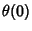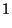(3)(4)

Solutionsfor, 1, 2, 3, and 4 are shown above. The cases, 1, and 5 can be solved analytically (Chandrasekhar 1967, p. 91); the others must be obtained numerically.

For(), the Lane-Emden Differential Equation is(5)

(Chandrasekhar 1967, pp. 91-92). Directly solving gives(6)(7)(8)(9)(10)(11)

The Boundary Condition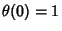then givesand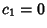, so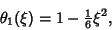(12)

andis Parabolic.

For(), the differential equation becomes(13)(14)

which is the Spherical Bessel Differential Equation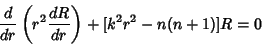(15)

withand, so the solution is(16)

Applying the Boundary Conditiongives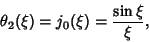(17)

where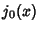is a Spherical Bessel Function of the First Kind (Chandrasekhar 1967, pp. 92).

For, make Emden's transformation(18)(19)

which reduces the Lane-Emden equation to(20)

(Chandrasekhar 1967, p. 90). After further manipulation (not reproduced here), the equation becomes(21)

and then, finally,(22)

References

Chandrasekhar, S. An Introduction to the Study of Stellar Structure. New York: Dover, pp. 84-182, 1967.